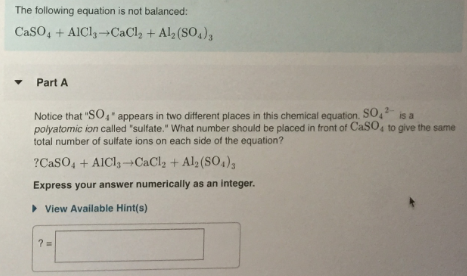# The following equation is not balanced: CaSO4 + AlCl3 → CaCl2 + Al2(SO4)3 Notice that "SO4" appears in two different places in this chemical equation. SO4^2- is a polyatomic ion called "sulfate." What number should be placed in front of CaSO4 to give the same total number of sulfate ions on each side of the equation? ?CaSO4 + AlCl3 → CaCl2 + Al2(SO4)3. Express your answer numerically as an integer,